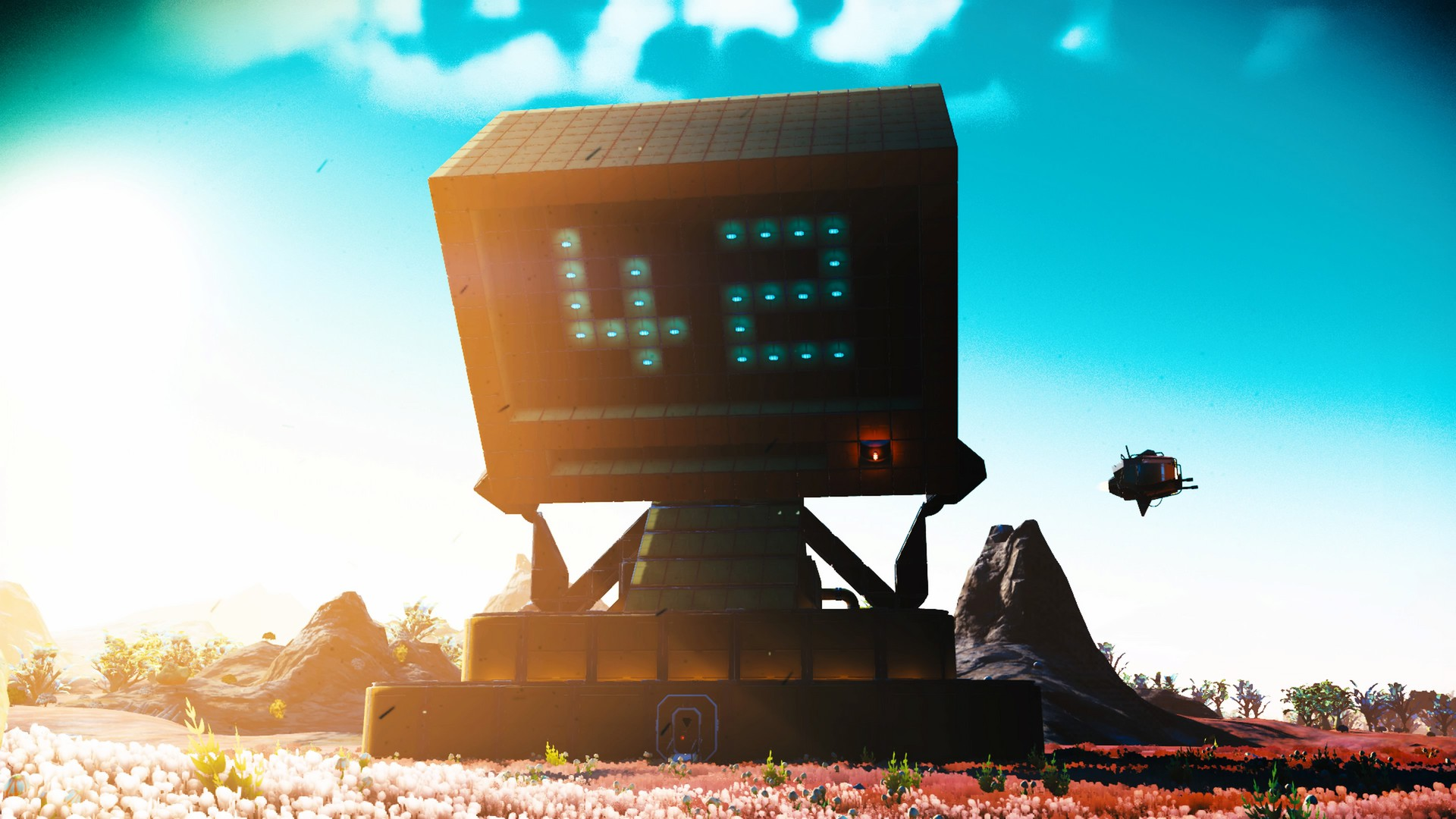# 42 is the sum of three cubesRobin Houston reports that 42 is the sum of three cubes. It's special in this regard not because it is alone, but because it was for an obvious reason the last remaining number under 100 where the sum was undiscovered.

42 = (-80538738812075974)^3 + 80435758145817515^3 + 12602123297335631^3

...

This computational breakthrough was achieved in a collaboration between Andrew Sutherland (MIT) and Andrew Booker (Bristol). They announced the result by both replacing their homepages with the expression – with the page title Life, the Universe and Everything.

Every cube of a whole number is within one of a multiple of nine, which means that a sum of three cubes must be within three of a multiple of nine. So numbers of the form 9𝑘+4 or 9𝑘+5 cannot be written as the sum of three cubes.

In 1992, Roger Heath-Brown conjectured that every other whole number can be written as the sum of three cubes, in infinitely many different ways. Mathematicians on the whole seem to have been convinced by Heath-Brown’s argument that this ought to be true – but actually finding ways to write any particular number as a sum of three cubes remains a difficult problem.